# MA.8.GR.1.1Export Print
Apply the Pythagorean Theorem to solve mathematical and real-world problems involving unknown side lengths in right triangles.

### Clarifications

Clarification 1: Instruction includes exploring right triangles with natural-number side lengths to illustrate the Pythagorean Theorem.

Clarification 2: Within this benchmark, the expectation is to memorize the Pythagorean Theorem.

Clarification 3: Radicands are limited to whole numbers up to 225.

General Information
Subject Area: Mathematics (B.E.S.T.)
Strand: Geometric Reasoning
Status: State Board Approved

## Benchmark Instructional Guide

### Terms from the K-12 Glossary

• Converse of Pythagorean Theorem
• Theorem

### Vertical Alignment

Previous Benchmarks

Next Benchmarks

### Purpose and Instructional Strategies

In grade 6, students worked with right triangles, with a focus on how the area of a right triangle is determined by its side lengths. In grade 8, students use the Pythagorean Theorem to determine side lengths of right triangles. In Geometry, students will use trigonometry to continue their work with right triangles, and they extend the understanding of the Pythagorean Theorem to create the equation of a circle.
• While it is not the expectation of this benchmark, instruction includes building understanding of the Pythagorean Theorem ($a$² + $b$² = $c$²) by proving it in various ways. Many of them connect the side lengths of right triangles to the areas of associated squares.
• Instruction includes using exploration activities that allow students to see how to use areas of squares to find missing sides. Students can use grid paper to draw right triangles from given measures and represent and compute the areas of the squares on each side. Below is an example of what students can visualize in order to understand the conceptual understanding within the Pythagorean Theorem.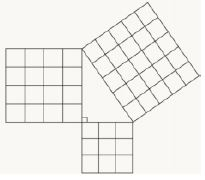• Data can be recorded in a chart such as the one below, allowing for students to conjecture about the relationship among the areas of squares and side lengths of right triangles.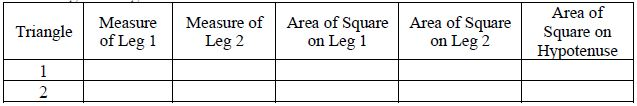• When introducing the Pythagorean Theorem, some students may not be able to visualize the side lengths and the connection to the values of $a$, $b$ and $c$. Using colors to color code the sides and hypotenuse will allow students to see the connection and identify with the $a$, $b$ and $c$ used to represent the sides.
• While solving real-world problems, students should be encouraged to draw diagrams where they can see the right triangles being used. Students will need to understand the ideas within the Triangle Inequality Theorem to help differentiate between the legs and the hypotenuse of a right triangle.

### Common Misconceptions or Errors

• Students may make errors in calculations when using the Pythagorean Theorem and finding square roots.
• Students may not be able to spatially visualize triangles within the real-world problems. To address this misconception, instruction includes models for these problems with triangles and drawings to help students orient the ideas within the tasks.
• Students may misidentify the side lengths and hypotenuse when connecting to the formula of $a$² + $b$² = $c$². To support students as they are developing the conceptual understanding of this benchmark, using the idea of $l$$e$$g$² + $l$$e$$g$² = $h$$y$$p$$o$$t$$e$$n$$u$$s$$e$² as a transition to using the formula.

### Strategies to Support Tiered Instruction

• Instruction includes modeling the differences between doubling and squaring a radius. Doubling a radius would be represented by multiplying the given length, whereas squaring a number would be represented by the area of a square with the given radius.
• For example, students can be given the table below to show how the left column doubles a length whereas the right column squares a length.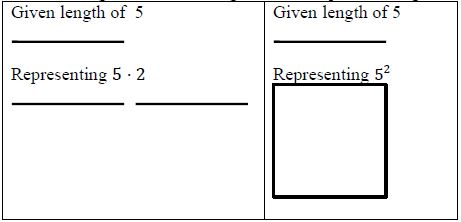• Teacher creates and posts an anchor chart for calculating the Pythagorean Theorem with visuals focused on solving for the variable and finding the square root.
• Instruction includes providing students with a right triangle as a visual in the context of a real-world problem. Teacher provides instruction using the information from the real-world problem to label the visual representation before solving.
• Instruction includes co-constructing a graphic organizer for the square root of perfect squares from 0 to 225 to provide students with the opportunity to determine benchmark numbers for non-perfect squares.
• Instruction includes color-coding and labeling a right triangle or a rectangular prism to provide a visual representation of variables, side lengths and hypotenuse.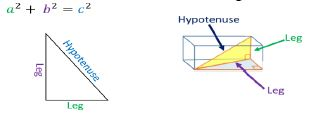• Instruction includes co-constructing a model with students and completing a graphic organizer to make the connection between the side lengths of right triangles to the area of the associated square.
• Instruction includes including time for reviewing solving for variables and finding square roots prior to instruction on this benchmark.
• Instruction includes models for problems with triangles and drawings to help students orient the ideas within the tasks.
• Instruction includes the use of the idea of $l$$e$$g$² + $l$$e$$g$² = $h$$y$$p$$o$$t$$e$$n$$u$$s$$e$² as a transition to using the formula to assist in developing a conceptual understanding of the benchmark for students that misidentify the side lengths and hypotenuse when connecting the formula of $a$² + $b$² = $c$² (laminating formulas on a printed card for students to utilize as a resource in and out of the classroom would be helpful).

Instructional Task 1 (MTR.2.1, MTR.4.1, MTR.7.1)
The bases on a baseball diamond are 90 feet apart on a standard baseball field.
• Part A. Draw a model of the baseball diamond.
• Part B. What is the distance, in feet, for the catcher to throw from home plate to second base?
• Part C. What is the distance, in feet, from first base to third base?

You are wrapping a gift for your teacher’s birthday. It is a very long and skinny pencil. You want to wrap it in a box so that your teacher cannot tell what shape it is. Your friend has a shoe box that measures 10 inches by 7 inches by 5 inches.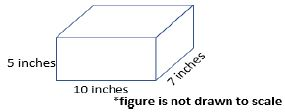• Part A. What questions would still need to be answered to approach this problem? Do you need all of the measurements provided in the problem? Explain your answer.
• Part B. If the pencil measures 13 inches long, will it fit in the shoe box with the lid closed? Explain your answer.
• Part C. What are the possible dimensions of a box that can just barely fit a pencil measuring 9 inches long?

### Instructional Items

Instructional Item 1
The bottom of a ladder must be placed 3 feet from a wall. The ladder is 10 feet long. How far above the ground does the ladder touch the wall?

Instructional Item 2
Using the figure below, find the value of the length of side $A$$B$ in meters.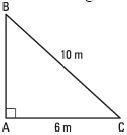Instructional Item 3
If a right triangle’s legs are both the same length, $x$, and the hypotenuse of the triangle is 25 feet, what is the value of $x$, in feet?

*The strategies, tasks and items included in the B1G-M are examples and should not be considered comprehensive.

## Related Courses

This benchmark is part of these courses.
1205050: M/J Accelerated Mathematics Grade 7 (Specifically in versions: 2014 - 2015, 2015 - 2020, 2020 - 2022, 2022 and beyond (current))
1205070: M/J Grade 8 Pre-Algebra (Specifically in versions: 2014 - 2015, 2015 - 2022, 2022 and beyond (current))
1204000: M/J Foundational Skills in Mathematics 6-8 (Specifically in versions: 2014 - 2015, 2015 - 2022, 2022 and beyond (current))
7812030: Access M/J Grade 8 Pre-Algebra (Specifically in versions: 2014 - 2015, 2015 - 2018, 2018 - 2019, 2019 - 2022, 2022 and beyond (current))

## Related Access Points

Alternate version of this benchmark for students with significant cognitive disabilities.
MA.8.GR.1.AP.1: Find the hypotenuse of a two-dimensional right triangle using the Pythagorean Theorem.

## Related Resources

Vetted resources educators can use to teach the concepts and skills in this benchmark.

## Formative Assessments

Three Dimensional Diagonal:

Students are asked to determine the length of a side of a right triangle in a real-world problem.

Type: Formative Assessment

Pythagorean Squares:

Students are asked to explain how a pair of figures demonstrates the Pythagorean Theorem and its converse.

Type: Formative Assessment

New Television:

Students are asked to determine the length of a side of a right triangle in a real-world problem.

Type: Formative Assessment

How Far to School:

Students are asked to determine the length of a side of a right triangle in a real-world problem.

Type: Formative Assessment

## Lesson Plans

Just Plane Ol' Area!:

Students will construct various figures on coordinate planes and calculate the perimeter and area. Use of the Pythagorean theorem will be required.

Type: Lesson Plan

Pythagoras - You Clever Dog:

This lesson starts with an introduction of the Pythagorean Theorem. It introduces vocabulary, formulas and concepts related to right triangles and the use of the Pythagorean Theorem in the real world. Students will learn the basics through real world application.

Type: Lesson Plan

## Original Student Tutorials

Pythagorean Theorem: Part 3:

Use the Pythagorean Theorem to find the legs of a right triangle in mathematical and real worlds contexts in this interactive tutorial.

This is part 3 in a 3-part series. Click below to explore the other tutorials in the series.

Type: Original Student Tutorial

Pythagorean Theorem: Part 2:

Use the Pythagorean Theorem to find the hypotenuse of a right triangle in mathematical and real worlds contexts in this interactive tutorial.

This is part 2 in a 3-part series. Click below to explore the other tutorials in the series.

Type: Original Student Tutorial

Pythagorean Theorem: Part 1:

Learn what the Pythagorean Theorem and its converse mean, and what Pythagorean Triples are in this interactive tutorial.

This is part 1 in a 3-part series. Click below to explore the other tutorials in the series.

Type: Original Student Tutorial

Applying the Pythagorean Theorem to Solve Mathematical and Real-World problems:

Apply the Pythagorean Theorem to solve mathematical and real-rorld problems in this interactive tutorial.

Type: Original Student Tutorial

## Perspectives Video: Expert

Measuring a Grid for Underwater Archeology:

Don't be a square! Learn about how even grids help archaeologists track provenience!

Type: Perspectives Video: Expert

## Perspectives Video: Teaching Idea

KROS Pacific Ocean Kayak Journey: Kites, Geometry, and Vectors:

Set sail with this math teacher as he explains how kites were used for lessons in the classroom.

Related Resources:
KROS Pacific Ocean Kayak Journey: GPS Data Set [.XLSX]
KROS Pacific Ocean Kayak Journey: Path Visualization for Google Earth [.KML]

Type: Perspectives Video: Teaching Idea

## Text Resource

Pythagoras Explained:

This informational text resource is intended to support reading in the content area. The text describes a method for predicting the win-loss record for baseball teams based on runs scored and runs allowed, using the "Pythagorean Expectation" formula invented by Bill James. The text goes on to show the relationship of the prediction formula to the Pythagorean theorem, pointing out a very cool application of the theorem to the world of sports.

Type: Text Resource

## MFAS Formative Assessments

How Far to School:

Students are asked to determine the length of a side of a right triangle in a real-world problem.

New Television:

Students are asked to determine the length of a side of a right triangle in a real-world problem.

Pythagorean Squares:

Students are asked to explain how a pair of figures demonstrates the Pythagorean Theorem and its converse.

Three Dimensional Diagonal:

Students are asked to determine the length of a side of a right triangle in a real-world problem.

## Original Student Tutorials Mathematics - Grades 6-8

Applying the Pythagorean Theorem to Solve Mathematical and Real-World problems:

Apply the Pythagorean Theorem to solve mathematical and real-rorld problems in this interactive tutorial.

Pythagorean Theorem: Part 1:

Learn what the Pythagorean Theorem and its converse mean, and what Pythagorean Triples are in this interactive tutorial.

This is part 1 in a 3-part series. Click below to explore the other tutorials in the series.

Pythagorean Theorem: Part 2:

Use the Pythagorean Theorem to find the hypotenuse of a right triangle in mathematical and real worlds contexts in this interactive tutorial.

This is part 2 in a 3-part series. Click below to explore the other tutorials in the series.

Pythagorean Theorem: Part 3:

Use the Pythagorean Theorem to find the legs of a right triangle in mathematical and real worlds contexts in this interactive tutorial.

This is part 3 in a 3-part series. Click below to explore the other tutorials in the series.

## Student Resources

Vetted resources students can use to learn the concepts and skills in this benchmark.

## Original Student Tutorials

Pythagorean Theorem: Part 3:

Use the Pythagorean Theorem to find the legs of a right triangle in mathematical and real worlds contexts in this interactive tutorial.

This is part 3 in a 3-part series. Click below to explore the other tutorials in the series.

Type: Original Student Tutorial

Pythagorean Theorem: Part 2:

Use the Pythagorean Theorem to find the hypotenuse of a right triangle in mathematical and real worlds contexts in this interactive tutorial.

This is part 2 in a 3-part series. Click below to explore the other tutorials in the series.

Type: Original Student Tutorial

Pythagorean Theorem: Part 1:

Learn what the Pythagorean Theorem and its converse mean, and what Pythagorean Triples are in this interactive tutorial.

This is part 1 in a 3-part series. Click below to explore the other tutorials in the series.

Type: Original Student Tutorial

Applying the Pythagorean Theorem to Solve Mathematical and Real-World problems:

Apply the Pythagorean Theorem to solve mathematical and real-rorld problems in this interactive tutorial.

Type: Original Student Tutorial

## Perspectives Video: Expert

Measuring a Grid for Underwater Archeology:

Don't be a square! Learn about how even grids help archaeologists track provenience!

Type: Perspectives Video: Expert

## Parent Resources

Vetted resources caregivers can use to help students learn the concepts and skills in this benchmark.

## Perspectives Video: Expert

Measuring a Grid for Underwater Archeology:

Don't be a square! Learn about how even grids help archaeologists track provenience!